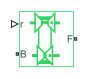# Variable Ratio Transmission

Dynamic gearbox with variable and controllable gear ratio, transmission compliance, and friction losses

• Library:
• Simscape / Driveline / Couplings & Drives

•## Description

The Variable Ratio Transmission block represents a gearbox that dynamically transfers motion and torque between the two connected driveshaft axes, the base and the follower.

When you choose to have the block ignore the dynamics of transmission compliance, it constrains the driveshafts to corotate with a variable gear ratio that you control. You can choose whether the follower axis rotates in the same or opposite direction as the base axis. If the follower and base axis rotate in the same direction, ωF and ωB have the same sign. If the follower and base axis rotate in opposite directions, ωF and ωB have opposite signs.

Transmission compliance introduces internal time delay between the axis motions. Unlike a gear, a variable ratio transmission does not act as a kinematic constraint. You can also control the torque loss caused by transmission and viscous losses.

### Ideal Motion and Torque Transfer

The Variable Ratio Transmission block dynamically transfers motion and torque between the base shaft and the follower shaft.

If the relative compliance ϕ between the axes is absent, the block is equivalent to a gear with a variable ratio gFB(t). Such a gear imposes a time-dependent kinematic constraint on the motions of the two driveshafts:

`${\omega }_{B}=±{g}_{FB}\left(t\right){\omega }_{F}$`
`${\tau }_{B}=±{g}_{FB}\left(t\right){\tau }_{F}$`

However, the Variable Ratio Transmission does include compliance between the axes. Dynamic motion and torque transfer replace the kinematic constraint, with a nonzero ϕ that dynamically responds through the base compliance parameters kp and kv:

`$\frac{d\varphi }{dt}=±{g}_{FB}\left(t\right){\omega }_{F}-{\omega }_{B},$`
`${\tau }_{B}=-{k}_{p}\varphi \left(t\right)-{k}_{v}\frac{d\varphi }{dt},$`
`$±{g}_{FB}\left(t\right){\tau }_{B}+{\tau }_{F}-{\tau }_{loss}=0.$`

τloss = 0 in the ideal case.

Estimating Compliance Parameters
• You can estimate the base angular compliance kp from the transmission time constant tc and inertia J.

`${k}_{p}=J{\left(\frac{2\pi }{{t}_{c}}\right)}^{2}$`
• You can estimate the base angular velocity compliance kv from the transmission time constant tc, inertia J, and damping coefficient C.

`${k}_{v}=2C\frac{{t}_{c}}{2\pi }=2C\sqrt{J}{{k}_{p}}}$`

### Nonideal Torque Transfer and Losses

With nonideal torque transfer, τloss ≠ 0. The Variable Ratio Transmission block models losses similarly to nonideal gears. For general information about nonideal gear modeling, see Model Gears with Losses.

In a nonideal gearbox, the angular velocity and compliance dynamics remain the same as in the ideal case. The transferred torque and power are reduced by:

• Coulomb friction, such as the friction between belt and wheel, or internal belt losses due to stretching, characterized by an efficiency η.

• Viscous coupling of driveshafts with bearings, parametrized by viscous friction coefficients μ.

`$\begin{array}{l}{\tau }_{loss}={\tau }_{Coul}\mathrm{tanh}\left(4{\omega }_{out}}{{\omega }_{th}}\right)+{\mu }_{B}{\omega }_{B}+{\mu }_{F}{\omega }_{F}\\ {\tau }_{Coul}=|{\tau }_{F}|\left(1-\eta \right)\end{array}$`

When the angular velocity changes sign, the hyperbolic tangent function smooths the change in the Coulomb friction torque.

Power FlowPower Loss Condition Output Driveshaft ωout
Forward ω B τ B > ω F τ F Follower, ωF
Reverse ω B τ B < ω F τ F Base, ωB

### Meshing Efficiency

The block only fully applies the friction loss represented by efficiency η if the absolute value of the follower angular velocity ωF is greater than a velocity threshold ωth.

If this absolute velocity is less than ωth, the block smooths the efficiency to one at zero velocity.

## Ports

### Input

expand all

Physical signal port associated with the input to output shaft ratio.

### Conserving

expand all

Mechanical rotational conserving port associated with the base driveshaft.

Mechanical rotational conserving port associated with the follower driveshaft.

## Parameters

expand all

### Main

Output driveshaft rotation relative to the input driveshaft.

### Compliance

Reciprocal of the transmission angular compliance kp measured at the base.

Reciprocal of the transmission angular compliance damping kv measured at the base.

Torque applied at the base driveshaft at the start of simulation.

### Transmission Losses

Implementation of friction losses from nonideal torque transfer.

• `No losses — Suitable for HIL simulation` — Torque transfer is ideal.

• `Constant efficiency` — The block reduces gear box torque transfer by a constant efficiency η satisfying 0 < η ≤ 1.

Effective torque transfer efficiency η between the base and follower driveshafts.

#### Dependencies

To enable this parameter, set Losses model to `Constant efficiency`.

Absolute angular velocity threshold ωth above which full efficiency loss is applied, for follower velocity ωF.

#### Dependencies

To enable this parameter, set Losses model to `Constant efficiency`.

### Viscous Losses

Vector of viscous damping coefficients [μB, μF] applied at the base and follower driveshafts, respectively.

## Version History

Introduced in R2011a